# GMAT Avengers Study Group: Coordinate Geometry:

by on October 6th, 2014

Coordinate geometry, or Cartesian geometry, is the study of geometry using a coordinate system and the principles of algebra and analysis.

## The Coordinate Plane

In coordinate geometry, points are placed on the “coordinate plane” as shown below. The coordinate plane is a two-dimensional surface on which we can plot points, lines and curves. It has two scales, called the x-axis and y-axis, at right angles to each other.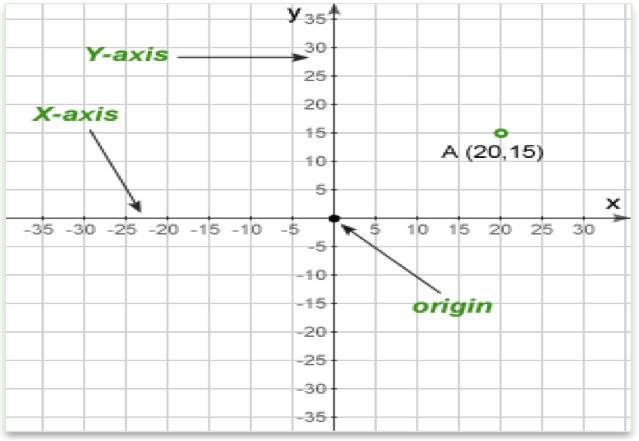In the diagram above, the point A has an x value of 20 and a y value of 15. These are the coordinates of the point A, sometimes referred to as its “rectangular coordinates.”

X axis – The horizontal scale is called the x-axis and is usually drawn with the zero point in the middle. Values to the right are positive and those to the left are negative.

Y axis – The vertical scale is called the y-axis and is also usually drawn with the zero point in the middle. Values above the origin are positive and those below are negative.

Origin – The point where the two axes cross (at zero on both scales) is called the origin.

Quadrants – When the origin is in the center of the plane, they divide it into four areas called quadrants.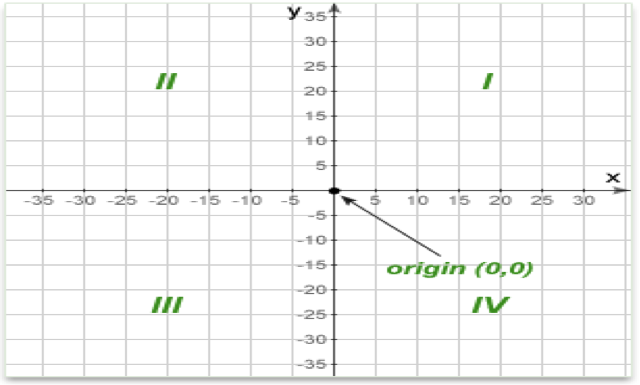In Quadrant I, x > 0 and y > 0

In Quadrant II, x < 0 and y > 0

In Quadrant III, x < 0 and y < 0

In Quadrant IV, x > 0 and y < 0

Point (x,y) – The coordinates are written as an “ordered pair.” The two numbers in parentheses are the x and y coordinate of the point. The first number (x) specifies how far along the x (horizontal) axis the point is. The second is the y coordinate and specifies how far up or down the y axis to go.

The sign of the coordinate is important. A positive number means to go to the right (x) or up (y). Negative numbers mean to go left (x) or down (y).

Distance between two points – the distance formula on the plane is derived from the Pythagorean theorem.

Above formula can be written in the following way for given two pointsand: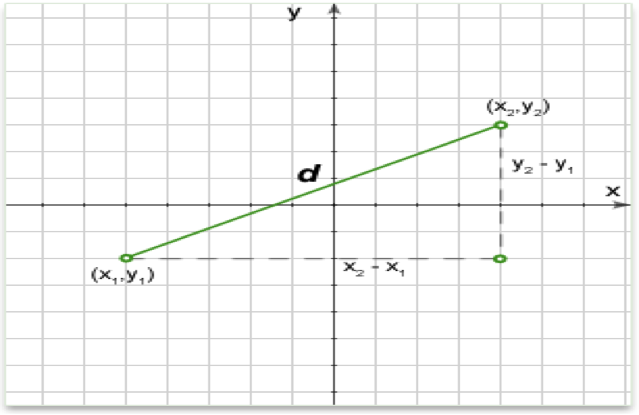Vertical and horizontal lines – If the line segment is exactly vertical or horizontal, the formula above will still work fine, but there is an easier way. For a horizontal line, its length is the difference between the x-coordinates. For a vertical line its length is the difference between the y-coordinates.

Distance between the point A (x,y) and the origin – As the one point is origin with coordinate O (0,0) the formula can be simplified to:

## Lines in Coordinate Geometry

In coordinate geometry, lines in a Cartesian plane can be described algebraically by linear equations and linear functions. Every straight line in the plane can represented by a first degree equation with two variables.

The following methods are used in order to define the line exactly:

1. General Form – The general form of the equation of a straight line iswhere a, b and c are arbitrary constants. This form includes all other forms as special cases. For an equation in this form the slope isand the y intercept is.

2. Point-intercept formThe standard equation here isWhere: m is the slope of the line;  b  is the y-intercept of the line; x is the independent variable of the function .

3. Using two points – The equation of a straight line passing through pointsandis:4. Using one point and the slope - Sometimes we will be given a point on the line and its slope and from this information we will need to find the equation or check if this line goes through another point.We can think of the slope as the direction of the line. So once we know that a line goes through a certain point, and which direction it is pointing, we have defined one unique line.

The equation of a straight line that passes through a pointwith a slope m is:5. Intercept formThe equation of a straight line whose x and y intercepts are a and b, respectively, is:## Slope of a Line

The slope or gradient of a line describes its steepness, incline, or grade. A higher slope value indicates a steeper incline.

The slope is defined as the ratio of the “rise” divided by the “run” between two points on a line, or in other words, the ratio of the altitude change to the horizontal distance between any two points on the line.

Given two pointsandon a line, the slope m of the line is:.

The slope of a line can be positive, negative, zero or undefined.

## Vertical and horizontal lines

A vertical line is parallel to the y-axis of the coordinate plane. All points on the line will have the same x-coordinate.

A vertical line has no slope. The equation of a vertical line is:, where: x is the coordinate of any point on the line; a is where the line crosses the x-axis (x intercept). Notice that the equation is independent of y. Any point on the vertical line satisfies the equation.

A horizontal line is parallel to the x-axis of the coordinate plane. All points on the line will have the same y-coordinate. A horizontal line has a slope of zero.

The equation of a horizontal line is:, where: x is the coordinate of any point on the line; b is where the line crosses the y-axis (y intercept). Notice that the equation is independent of x. Any point on the horizontal line satisfies the equation

Parallel Lines – Parallel lines have the same slope. We can calculate the slope from the following:

• From two given points on the line.
• From the equation of the line in slope-intercept form
• From the equation of the line in point-slope form

The equation of a line through the pointand parallel to lineis:Perpendicular lines – For one line to be perpendicular to another, the relationship between their slopes has to be negative reciprocal. In other words, the two lines are perpendicular if and only if the product of their slopes is -1.

Intersection of two straight lines – The point of intersection of two non-parallel lines can be found from the equations of the two lines.

To find the intersection of two straight lines, we need the following :

• The respective equations of the lines.
• Then, since at the point of intersection, the two equations will share a point and thus have the same values of x and y, we set the two equations equal to each other. This gives an equation that we can solve for x.
•  We substitute the x value in one of the line equations (it doesn’t matter which) and solve it for y.

If both lines are vertical or horizontal, they are parallel and have no intersection

Circle on a plane – In an x-y Cartesian coordinate system, the circle with center (a, b) and radius r is the set of all points (x, y) such that:This equation of the circle follows from the Pythagorean theorem applied to any point on the circle: as shown in the diagram above, the radius is the hypotenuse of a right-angled triangle whose other sides are of lengthand.

Number line – A number line is a picture of a straight line on which every point corresponds to a real number and every real number to a point

Parabola – A parabola is the graph associated with a quadratic function, i.e. a function of the form:.

Let’s now talk about the articles that we share on the Facebook Event Page. Here’s a quick recap of them:

## Problem Solving Practice

1) Consider the three points in the x-y plane: P = (8, 4), Q = (6, 7), and R = (9, 0). Rank these three points from closest to the origin, (0, 0), to furthest from the origin.

(A) P, Q, R

(B) P, R, Q

(C) Q, P, R

(D) R, P, Q

(E) R, Q, P

2) Trapezoid JKLM in the x-y plane has coordinates J = (–2, –4), K = (–2, 1), L = (6, 7), and M = (6, –4). What is its perimeter ?

(A) 34

(B) 36

(C) 38

(D) 40

(E) 42

3) A certain square is to be drawn on a coordinate plane. One of the vertices must be on the origin, and the square is to have an area of 100. If all coordinates of the vertices must be integers, how many different ways can this square be drawn?

(A) 4

(B) 6

(C) 8

(D) 10

(E) 12

4) The center of circle Q is on the y-axis, and the circle passes through points (0, 7) and (0, –1). Circle Q intersects the positive x-axis at (p, 0). What is the value of p?

(A) 7/3

(B) 4

(C) 5

(D)(E)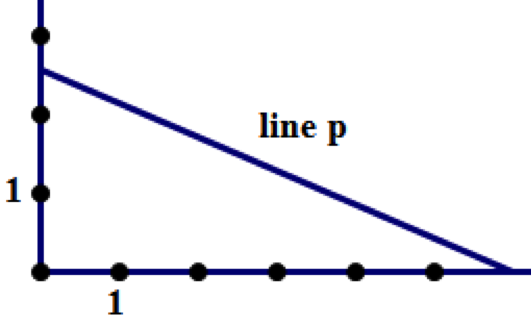5) In the coordinate system above, which of the following is the equation of line p?

(A)(B)(C)(D)(E)6) What is the area of a triangle created by the intersections of the lines x=4, y=5 and?

(A) 42

(B) 54

(C) 66

(D) 72

(E) 96

7) Line l is defined by the equationand line w is defined by the equation. If line k does not intersect line l, what is the degree measure of the angle formed by line k and line w? (Assume that all lines lie in one coordinate plane.)

(A) 0

(B) 30

(C) 60

(D) 90

(E) It cannot be determined from the information given

## Data Sufficiency Practice

1) Triangle RST lies in the xy-plane. Point R has coordinates (a,b), point S is at the origin, and point T has coordinates (c,d), such that. What is the area of triangle RST?

(A)(B)2) In the xy-plane, region Q consists of all points (x, y) such that≤ 100. Is the point (a, b) in region Q?

(A) a + b = 14

(B) a > b

3) In the xy-plane, does the line with equationcontain the point (r, s)?

(A)(B)4) If x and y are both positive, is x greater than 4?

(A) x > y

(B) In the coordinate plane, the point (x, y) lies outside a circle of radius 5 centered at the origin.

5) In the x-y coordinate plane, what is the minimum distance between a point on line L and a point on line M?

(A) The absolute value of the difference between the y-intercepts of the two lines is 4.

(B) The absolute values of the slopes of the two lines are both equal to 2.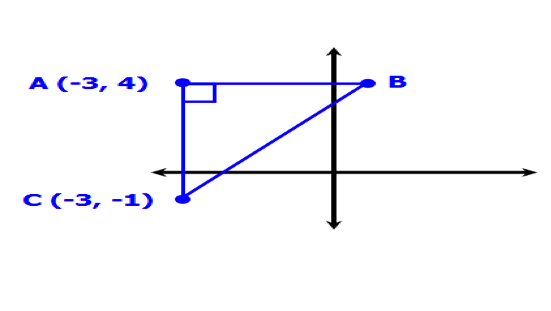6) What are the coordinates of point B in the figure above ?

(A) The area of ∆ABC = 30

(B) Length of CB = 13

7) In the xy-plane region R consists of all the points (x,y) such that<6. Is the point (r, s) in region R?

(A)(B) r < 3 and s < 2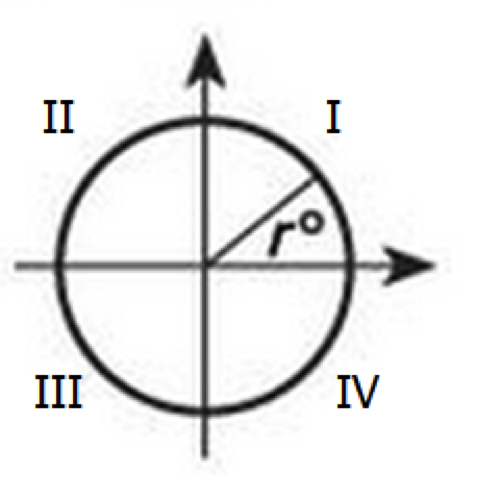(A) In each 30-second period, the beam sweeps through 3690°

(B) r = 40

9) Is the point (x, y) in the fourth quadrant?

(A) xy > 0

(B) y > 0

Problem Solving

1. B
2. A
3. E
4. D
5. A
6. E
7. D

Data Sufficiency

1. A. Statement (1) ALONE is sufficient, but statement (2) alone is not sufficient
2. E. Statements (1) and (2) TOGETHER are NOT sufficient
3. C. BOTH statements TOGETHER are sufficient, but NEITHER statement ALONE is sufficient
4. E. Statements (1) and (2) TOGETHER are NOT sufficient
5. E. Statements (1) and (2) TOGETHER are NOT sufficient
6. D. EACH statement ALONE is sufficient
7. E. Statements (1) and (2) TOGETHER are NOT sufficient
8. A. Statement (1) ALONE is sufficient, but statement (2) alone is not sufficient
9. D. EACH statement ALONE is sufficient

Make sure you check out the event Facebook page to see all the comments that were being exchanged during the live session.

***

EXCITING NEWS!! “The GMAT Avengers Guide” is now available! This eBook is a collection of transcripts, tips, strategies, recommended readings, best practices, and challenge problems to help you beat the GMAT and achieve your dream score. Download your FREE copy NOW!

RSVP NOW if you want to join me and fellow GMAT Avengers take on Volume questions.

•Data Sufficiency Q7 - Is there any algebraic solution to the problem?.
I picked up some numbers to test the conditions and got answer C

Data Sufficiency Q8 is missing.

Data Sufficiency Q9. Shouldn't the answer be (B).
For condition A, both points can be in First quadrant too. Hence insufficient.

•solutions for the problems??